Can you determine whether the two cars will collide?

Okay, get calculating. Can you do it?

Hey, did you hear the story about the guy (Roger) that watched his friend pilot a little propeller-driven Cessna over the course of several days? From the front passenger seat, he studied his friend carefully; the mechanics of flying all made sense. Then, one day, they took to the sky and the friend had a seizure. Roger grabbed the controls and carefully landed the planet. Sound plausible? There's no substitute for practice.

A little math is required to determine if the two cars collide. (Unless you just wait and see!) Most of us take the need for mathematics for granted; of course we need math to answer this question! But why? And what does this say about our universe? It's worth considering for a moment.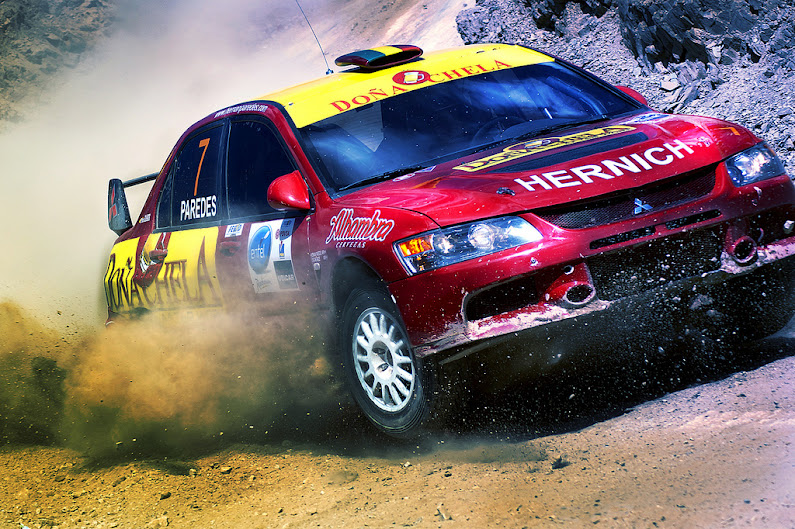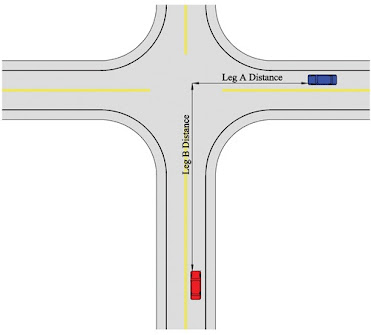All things in the whole wide world happen mathematically. -- Gottfried Wilhelm Leibniz

How is it possible that mathematics, a product of human thought that is independent of experience, fits so excellently the objects of reality? -- Albert Einstein

The most distinct and beautiful statements of any truth must take at last the mathematical form.

-- Henry David Thoreau

The miracle of the appropriateness of the language of mathematics for the formulation of the laws of physics is a wonderful gift which we neither understand nor deserve. We should be grateful for it and hope that it will remain valid in future research and that it will extend, for better or for worse, to our pleasure, even though perhaps also to our bafflement, to wide branches of learning.

-- Eugene Wigner

Ordinary language is totally unsuited for expressing what physics really asserts, since the words of everyday life are not sufficiently abstract. Only mathematics and mathematical logic can say as little as the physicist means to say.
-- Bertrand Russell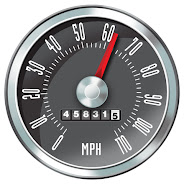A car's speedometer measures its instantaneous speed.Can a car's average speed ever exceed its instantaneous speed?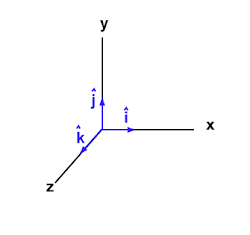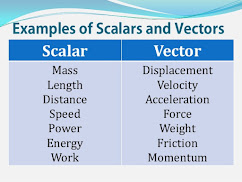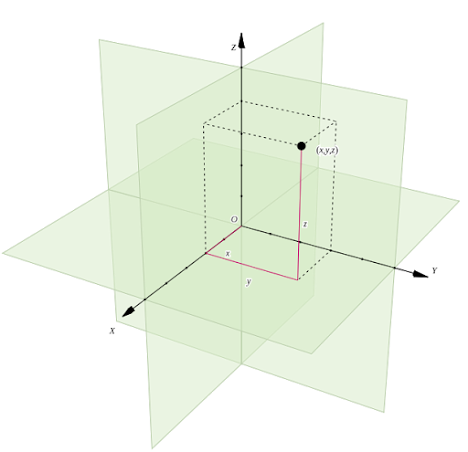Let's get to the math. It comes down to a single equation; actually, no more than a definition.

average speed = distance / time

The time for the red car to reach the intersection is: time = distance / average speed = [200.0 m] / [26.8 m/s] = 7.46 s. The time for the blue car to reach the intersection is: time = [100.0 m] / [20.1 m/s] = 4.98 s. The cars reach the intersection at different times, so no, the cars do not collide.

Were you bothered by the "average" in average speed? It implies a period of time over which speed might vary. If the red car were to speed up, from 20 m/s to 40 m/s, as it approached the intersection, the car's average speed would be 30 m/s. Substituting that number into the equation would result in a calculated time of 6.67 s for the red car to reach the intersection. Yes, this simple equation can handle (steady) acceleration.

We will typically wish to differentiate between an object's speed at some time, something we call instantaneous speed, and its average speed over some length of time. From the more recent example, when the red car reached the intersection, its instantaneous speed was 40 m/s, while its average speed was 30 m/s. If an object maintains a constant speed, its instantaneous and average speeds are identical at all times. This was the case for the red car in the original scenario.

1. A car steadily (uniformly) accelerates from 15 m/s to 35 m/s over a period of 3.0 seconds. How far does it travel during this time?

One of the primary objectives of a physicist or

engineer is to create or fine-tune a model for

some part of the world. The purpose of the model

may be to better understand that small part of

the world, or to predict its future state, or it may

be more practical, e.g. modeling a new and

improved braking system for a car, which is going

to be manufactured and placed in next year's model.

In order to model something that is moving,

you need to know its speed, and you need to

know if that speed is changing (and, if it is, how

quickly it's changing), but you also need to know

the direction the thing is moving.

Thus, physicists and engineers almost always speak of an object's velocity, a quantity that indicates both speed and direction. Using velocity instead of speed makes for a better model, better in the sense that it is more useful.

Study the following examples to see how direction is typically indicated when describing motion.

30 m/s east or right : 30 i (m/s)

30 m/s west or left : -30 (m/s)

50 m/s north or up : 50 (m/s)

50 m/s south or down : -50 (m/s)

m/s at 45° north of east: 1 i +1 (m/s)

(If the last example does not make sense to you, don't worry.

If it does, you're ahead of the game.)

Any quantity that can be measured and that includes (or could

include) a direction, like velocity, is called a vector quantity.

Acceleration is also a vector quantity, because when you

accelerate, you do so in a particular direction. Temperature is NOT

a vector quantity; rather, it is a scalar quantity. A scalar is a measurement without direction. The table at left includes other examples.

When we model motion, we imagine it happening within a Cartesian coordinate system. An object moves from one point within the coordinate system, specified by coordinates (x1,y1) or perhaps (x1,y1,z1), to another point, (x2,y2) or perhaps (x2,y2,z2). (Note: We will restrict ourselves to 2-dimensional motion in this unit.)

Let's say a ladybug is walking along the x-axis of our coordinate

system. It begins at (5,0) and walks to (8,0).

It's change in position, defined as

displacement (Δx), is written as:

Δx = 8 i - 5 i = 3 i (cm, or whatever units

we happen to be using to measure distance)

Notice that Δx = xf - xi; i.e. final position minus

initial position.

Motion along the x-axis, as you see, is

identified by the letter i. (Later on, we'll examine

the significance of i more deeply.) Motion along

the y-axis is identified by j, and we use k for

the z-axis.

This may all seem very unusual and

unnecessary, but trust me when I say that it

becomes very useful when modeling complicated

motion. And it's really not so difficult to become

familiar and comfortable with the notation.

First, a definition:

average velocity = displacement / time

In variable form:

vavg = Δx / t

Now, imagine that ladybug moves from (8,0)

to (20,0), with distance measured in centimeters,

in a time of 10 seconds. What is the ladybug's average velocity

vavg = (20 i - 8 i) / 10 = 12 i / 10 = 1.2 i (cm/s)

What if the ladybug moved from (0,5) to (0,15) in 20 seconds? What would its average velocity be?

vavg = (15 j - 5 j) / 20 = 10 j / 20 = 0.5 j (cm/s)

We've spoken of acceleration. Let's formally define it.

acceleration = change in velocity / time   or    a = Δv / t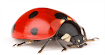Let Vi and Vf represent the initial and final velocities of an object, respectively.

vavg = (vi + vf) / 2

Δv = vf - viRené Descartes (1596-1650), French philosopher, scientist, mathematician, and the inventor of the coordinate system which bears his name.

Descartes is perhaps most famous for his philosophical conclusion: "I think, therefore I am."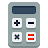Whether calculating Δx or Δv or the change in any other variable, always subtract the initial value from the final value.

Okay, so imagine the ladybug now accelerates from 1.5 i (cm/s) to 2.5 i (cm/s) in a time of 4.0 seconds.

vavg = (1.5 i + 2.5 i) / 2 = 2.0 i (cm/s)

Δv = 2.5 i - 1.5 i = 1.0 i (cm/s)

a = Δv / t = 1.0 i / 4.0 = 0.25 i (cm/s/s)

Also, given that vavg = Δx / t, which rearranges to Δx = vavg t, we can calculate that the ladybug's displacement during the 4.0 seconds was Δx = (2.0 i) 4.0 = 8.0 i (cm).

Practice the equations above by solving the following problems.

1. A car accelerates from 6.0 j (m/s) to 20.0 j (m/s) in a time of 3.5 seconds. Find the car's displacement and acceleration.
2. A car accelerates from -10.0 i (m/s) to -32.0 i (m/s) in a time of 2.5 seconds. Find the car's displacement and acceleration.

​The answers to the questions above can be found by placing your mouse here:

(Solve the problems before checking! Remember Roger.)

A bit of mathematical re-arrangement gives us the following useful equations.

a = Δv / t        thus        Δv = at        thus        vf - vi = at        thus        vf = at + vi

and

Δx = vavgt        thus        xf - xi = vavgt        thus        xf = vavgt + xi

Realize that these two highlighted equations are really nothing more than definitions of acceleration and average velocity. While they alone are sufficient to model objects that move in straight lines with a constant acceleration (including no acceleration), they may be mathematically combined to generate two new equations that, while saying nothing new, are useful to know. Let's do that:

Δx = vavgt        thus        Δx = ½ (vi + vf)t        thus        Δx = ½ (vi + at + vi)t        thus        Δx = ½ (2vi + at)t

which simplifies to        Δx = vit + ½ at2                         sometimes written as xf = xi + vit + ½ at2

Finally, vf - vi = at        leads to        t = (vf - vi) / a

and Δx = ½ (vi + vf)t        thus        Δx = ½ (vi + vf)(vf - vi) / a        thus        2aΔx = (vi + vf)(vf - vi)

thus        2aΔx = -vi2 + vf2        thus        vf2 = vi2 + 2aΔx

Δx = vavgt                       Δx = vit + ½ at2

vf = at + vi                       vf2 = vi2 + 2aΔx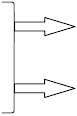The standard 4

kinematic equations.

(The 2 on the right are

derived from the 2 on the

left.)

The best thing we can do now is to practice keeping track of moving objects using the 4 equations above.

As we do so, we will follow the following basic  problem-solving guidelines:

First, read and fully comprehend what is physically happening in the "problem". Draw a picture of it, if that helps. Decide what it is that you're trying to determine.

Second, write down and label the numerical values given to you. If the values are vector quantities, with an associated direction, write down the direction, as well.

Third, decide which of the 4 standard kinematic equations is best for modeling the situation. Basically, pick out an equation for which you have values for all of the variables except the one that you are solving for.

Fourth, algebraically rearrange the kinematic equation, solving for the variable that you are trying to determine.

Fifth, substitute numbers into the equation, making sure that units are consistent. If units are not consistent, e.g. one variable is in terms of meters and another centimeters, convert units as necessary so that they are consistent. Then calculate your answer.

Sixth, clearly write down and label your answer, including proper units and direction (if applicable). If you can, try to gauge if the answer sounds reasonable. If it does not, check your work for mistakes.

Example 1

A car is heading north at 22.4 m/s (about 50 mph), whereupon it begins to accelerate at a constant 4.0 m/s2 in the same direction. What is the car's displacement in 6.0 seconds?

First, we have a car accelerating forward, and we want to know how far it travels in a time of 6 seconds. Specifically, we want to calculate Δx, the car's displacement. We don't need its current Cartesian coordinates or future Cartesian coordinates; we just need the difference between them. That's good, because we don't know enough to be able to pinpoint the actual coordinates. Because the car is heading north, it is aligned with the y-axis and we'll indicate its direction with j.

Second, here's what we know: vi = 22.4 j (m/s), a = 4.0 j (m/s2), t = 6.0 (s)

Third, we choose the equation Δx = vit + ½ at2, because it contains the variables we know plus the one variable we're solving for.

Fourth, ... well, the equation is already arranged to obtain Δx, so we don't need to rearrange it.

Fifth, we substitute numbers into the equation. Δx = (22.4 j)(6.0) + ½ (4.0 j)(6.02) = 206.4 j (m)

Sixth, our answer is: Δx = 206.4 j (m). That is, the car moves 206.4 meters north. Realize that Δx indicates a displacement and not the direction of the displacement; i.e. we are not aligned with the x-axis just because we're writing Δx. The j indicates the direction. (Note: The answer does not have the proper number of significant digits, but we're not going to worry about that.)

Example 2

A car is driving south at 5.0 m/s and accelerates to 25 m/s (still south) in a time of 3.0 seconds. What is the car's rate of acceleration?

I did step one. Did you? And we're trying to find a.

vi = -5.0 j (m/s), vf = -25 j (m/s), t = 3.0 (s)

vf = at + vi

a = (vf - vi) / t

a = (-25 j - -5.0 j) / 3.0 = (-25 j + 5 j) / 3.0 = (-20 j) / 3.0 = -6.7 j (m/s2)

a = -6.7 j (m/s2) : The car's acceleration is 6.7 m/s2 southward.

Example 3

A car is going 26 m/s to the right. If it's capable of slowing at the rate of 3.5 m/s2, then how much space does it need to come to a complete stop?

We're trying to find Δx.

vi = 26 i (m/s), vf = 0 (m/s), a = -3.5 i (m/s2)

That the final velocity was 0 was implied by the phrase "come to a complete stop". Look out for implied data. Also, it's not necessary to associate a direction with a vector quantity that is zero.

Why make the acceleration negative? Because an acceleration in the same direction as the velocity will cause the object to speed up, while an acceleration in the opposite direction will cause the object to slow down. Remember this!

vf2 = vi2 + 2aΔx

Δx = (vf2 - vi2) / 2a

Δx = (02 - 262 i) / 2(-3.5 i) = -676 i / -7.0 i = 96.6 i (m)

The car needs 96.6 meters in which to come to a stop.

Example 4

A rocket in outer space accelerates "to the left" at 18 m/s2 for 30 seconds. Following the acceleration, the rocket is traveling at 490 m/s "leftward". What was its initial velocity? (I put "leftward" in quotes because there is no well-defined left and right, or up and down, in space.)

We're trying to find vi.

a = -18 i (m/s2), vf = -490 i (m/s), t = 30 (s)

vf = at + vi

vi = vf - at

vi = (-490 i) - (-18 i)(30) = 50 i (m/s)

The rocket was initially traveling at 50 m/s to the right.

Example 5

A car accelerates steadily from 4.2 m/s to 8.5 m/s in a time of 0.88 seconds. How far did the car travel during this time?

No direction is specified. In such cases, we act as if the motion is happening along a particular axis (let's say the x-axis) and solve the problem as normal. When we state our answer, we simply do not state the associated direction. And if you look at how the problem is phrased -- "How far did the car travel..." -- we are being asked to calculate a distance (which is a scalar quantity), not a displacement with direction.

We are looking for Δx, excluding direction.

Let's assume rightward motion along the x-axis, just to help us solve the problem.

vi = 4.2 i (m/s), vf = 8.5 i (m/s), t = 0.88 (s)

So which equation to use? Recalling that vavg = (vi + vf) / 2, we have what we need to use the equation Δx = vavgt.

Δx = (4.2 i + 8.5 i)(0.88) / 2 = 5.6 i (m)

I'm sure you noticed that failure to include the i's does not affect your calculation. It's true, and many physicists will leave them out when dealing with one-dimensional motion. But do realize that things that are leftward or downward do require a negative sign.

The car traveled 5.6 meters.

Example 6

A jetliner, traveling northward, is landing with a speed of 58 m/s. Once the jet touches down, it has 650 m of runway in which to reduce its speed to 6.1 m/s. Calculate the necessary acceleration of the jetliner during this period.

We're trying to find a. Will the acceleration be positive or negative? Think about it before moving on.

vi = 58 j (m/s), vf = 6.1 j (m/s), Δx = 650 j (m)

vf2 = vi2 + 2aΔx

a = (vf2 - vi2) / 2Δx

Because this is one-dimensional motion, we're going to leave off the j's. We'll add one to the answer, though.

= (6.12 - 582) / 2(650) = -2.6 (m/s2)

The acceleration is -2.6 j (m/s2) or 2.6 m/s2 southward.

Example 7

The three-toed sloth is the slowest moving land animal. On the ground, the sloth moves with an average speed of 0.037 m/s, considerably slower than the giant tortoise, which walks at 0.076 m/s. After 12 minutes of walking, how much further would the tortoise have gone relative to the sloth?

This problem is not difficult, but you might not initially know how to work out the answer. (If you do, I could easily find a problem that you'd struggle with.) Working out how to set up a problem is a completely different task than performing a calculation. Many people, by the time they take physics in high school, are fairly good at calculations. But few people are initially good at setting up problems, which is a necessary first step. Taking a physics course is a chance to build this useful skill. Do not be frustrated if you struggle to set up problems. That's completely normal, and your goal should be to get better at it by watching others that can already do it and through practice.

Okay, so we have two things that are moving at different speeds. They will thus cover different distances in a common period of time. We want to find the difference between these two distances.

Typically, when you have two independent objects that are moving, you model them separately. Later, you may seek a way to combine the models, and that's what we'll do.

We want to find the Δx of the sloth. We already know that its vavg = 0.037 m/s and the time its moving is t = 12 min. As in Example 5, a direction is not specified, so we'll handle the problem in the same way. We'll assume motion along the x-axis, realizing that it doesn't matter. And we won't specify a direction in our answer. We'll also assume one-dimensional motion. If it's unclear why, think carefully about it.

The kinematic equation Δx = vavgt is a useful model; it has what we need. And it's already arranged as we need it.

It's time to substitute numbers into this equation, but here we have a situation in which units are not consistent. The speed refers to seconds and the time to minutes. So, we'll convert 12 minutes into some number of seconds -- it comes out to 720 s -- and we'll substitute time in seconds into the equation. Yes, we could have converted the seconds embedded in the speed into minutes, and we'd still have arrived at the same final answer. However, it's typically better to convert to seconds. You should be able to figure out why over the course of the year. (Always remember to check for unit consistency!) For the sloth:

Δx = (0.037 i)(720) = 26.64 i (m)

Similarly, we can model the motion of the tortoise.

Δx = (0.076 i)(720) = 54.72 i (m)

Neither of these displacements is what we're after. Rather, we want the difference between them, and we don't care about the direction, which was arbitrarily assumed anyway.

The difference between these two displacements is 54.72 i - 26.64 i = 28.08 i (m). That is, the tortoise travels 28.08 meters farther than the sloth during the 12 minutes.

One last note: Yes, I made this problem seem long. We talked through it conceptually. But once you "get it", you can knock out a problem like this in less than a minute.

Δx = (0.037 i)(720) = 26.64 i (m)The units for acceleration are of the form cm/s/s [read centimeters per second per second], typically abbreviated cm/s2. An acceleration of 5 cm/s/s means that speed is increasing 5 cm/s every second.The kinematic equations are easily derived using calculus. Click below to see how.The x-y plane can be arranged vertically (as you see up next to the compass) or horizontally (as you see directly above). There is no correct arrangement. Select whichever arrangement you want, unless you are solving a textbook problem and the author has selected an arrangement for you. Then use that one.Look carefully at Example 2. Does a negative acceleration mean that you are slowing down?In everyday language, to accelerate means to "speed up". Not so in physics. Here, to accelerate simply means to change your velocity.

If the direction of the acceleration matches the direction of velocity, the object will "speed up", while if the direction of acceleration is opposite to that of the velocity, the object will "slow down".

While an object always travels in the direction of its velocity, it does NOT always travel in the direction of its acceleration. Specifically, it does not when it's slowing down.A vector, as you'll recall, has two parts: the number part, called the magnitude, and  the direction.

In the example of a = -6.7 j (m/s2), the magnitude of the acceleration is 6.7 m/s2, and the direction is - j.practice  problems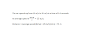-the Physics Classroom website (many pages on 1-D Kinematics, not all of which you're ready for)

-CK-12 online textbook (Chapter 2: One-Dimensional Motion)